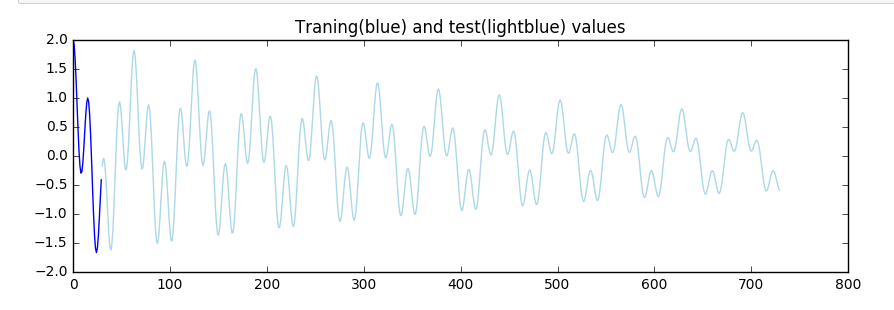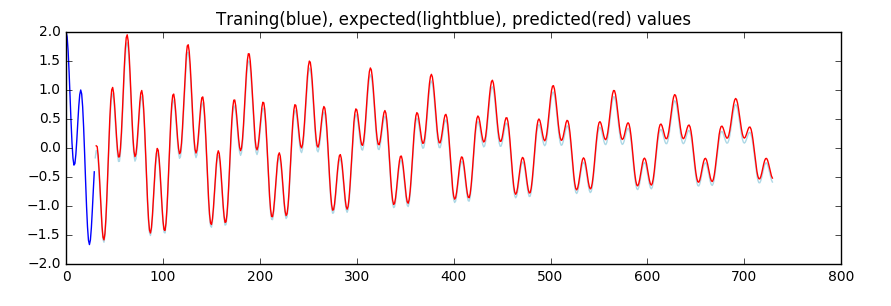# ML: Predict sequence of values

Let's say we have nice a line built up of two damped oscillators as it displayed on the picture.

What if I say it's possible to predict 700 values of this line using just:

• 30 data points for feeding neural network(which is just half-period)
• using just two fully-connected layers (hence it's not deep network)
• having just three neurons in whole network

I was quite surprised how fast NN could learn from such a small input even without "seeing" one full period. In previous experiment I trained network providing actual argument of that function as input of NN.

So what is the difference? In this experiment you can find method dataset() which generates pair of (X, Y) from the actual function values. It's something similar to "sliding window" over function's values. For instance if our function f() produces values (n1, n2, n3, n4) then dataset to be fed to NN looks like (n1, n2), (n2, n3), (n3, n4).

## Show me output!Red line is what our NN has predicted. Barely visible light blue spikes - true value. Not bad for just 3 neurons, huh?

In the first cell of notebook you can play with hyperparameters (SAMPLES, EPOCHS, BATCH_SIZE) and see differences in the result. Default ones aren't optimal thats why you can spot light blue line on the chart. Increasing amount of input datapoints and/or number of epochs will dramatically increase precision quality and MSE → 0

Open Experiment In GitHub

For other experiments using keras check out this 1st post